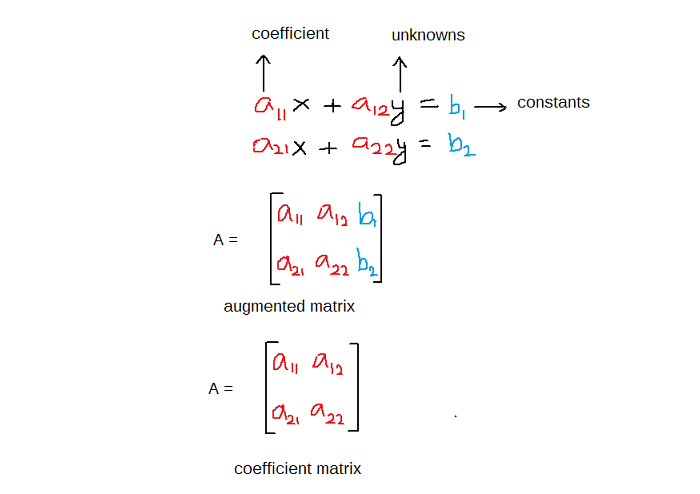# Solving System Of Linear Equations Using Cramer’s Rule

Cramer’s rule is a method of solving the system of linear equations using determinants. It is named after Gabriel Cramer (1704–1752) who discovered this method. In this article we are going to discuss and work few examples for solving system of linear equations using Cramer’s rule.

Before you start reading about Cramer’s rule, learn to compute determinant of 2 x 2 and 3 x 3 Matrix as a prerequisite to this article : Finding Determinants Using Cross Multiplication.

Coefficient Matrix and Augmented Matrix

Given a system of equation you can derive a coefficient matrix and an Augmented Matrix from it. Both are the same thing with little different.

Suppose you are given a system of linear equations with 2 unknowns.

The augmented matrix and coefficient matrix would look like the following.The system of linear equation is in the form of which is written as

The matrix is coefficient matrix, is a column vector of unknowns, and represents the constant vector.

Determinant Of 2 x 2 Matrix

The determinant of a 2 x 2 matrix can be calculated using cross multiplication.

Example #1

Find the determinant of .

Solution:

### Solving System of Equations with 2 Unknowns With Cramer’s Rule

Given a system of equations with 2 unknowns you can solve it using Cramer’s rule by following steps.

1. Find the augmented matrix

The post Solving System Of Linear Equations Using Cramer’s Rule appeared first on Notesformsc.

This post first appeared on Notesformsc, please read the originial post: here

# Share the post

Solving System Of Linear Equations Using Cramer’s Rule

×